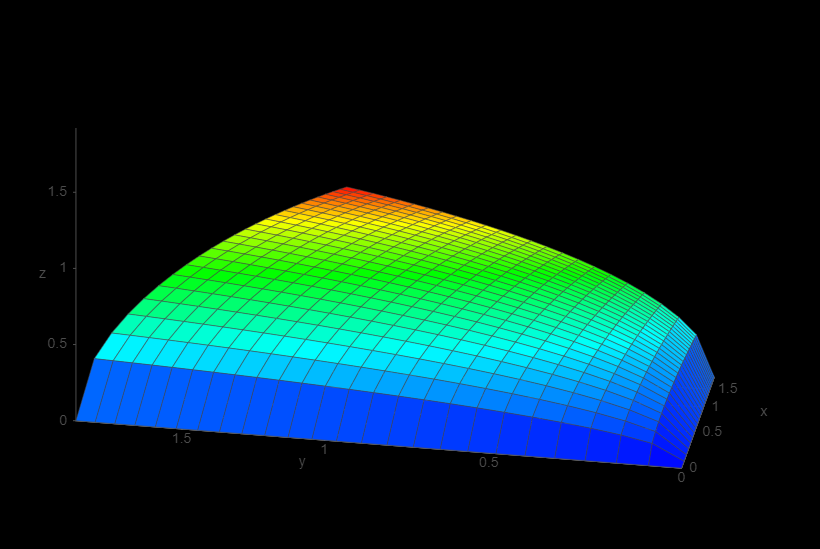top of page# Thoughts On Combo Correctives

How should you drive combo corrective blendShapes? Consider the following options:

### weight_1* weight_2 = combo_weight

Before I started working at Boulder Media I would drive combo corrective blendShapes by multiplying the weight of the two targets the combo was correcting. If we graph the driver function with the combo weight as the height of the graph we can visually see how the driver works.As we increase either weight_1 or weight_2 the combo weight increases linearly (which is desired result).However,when we increase them both at the same time we get something a bit odd. If weight_1 is the blendShape for moving the mouthCorner out and weight_2 is moving the mouthCorner up, then when we move it up and out at the same time with this combo driver it would increase slowly, reach a value of 1 when weight_1 and weight_2 are 1 and then it increases quickly so when weight_1 and weight_2 are both 2 the combo blendShape is 4, not 2 like we would want. Because the combo weight is increasing more quickly than the individual weights the blendShapes "blow up".

### (weight_1* weight_2)^.5 = combo_weight

When I started at boulder one of the riggers told me that taking the square root after multiplying weight_1 and weight_2 was a better way to drive the combo weights. It made sense to me at the time but it does have issues.So with this new function we can see that when we increase weight_1 and weight_2 at the same time the combo weight increases linearly which is what we want. When weight_1 and weight_2 are 2 the combo is also 2, so the blendShape doesn't "blow up".However when we have one weight already at a value greater than 0 and then start to increase the other weight the combo weights will jump up quickly at the start and then increase very slowly. This quick increase in the combo weights can be seen in the steep slope of the graph, and it will cause the blendShape to "pop" as it suddenly increases in weight.

So is there a functions that, like Hannah Montana, gets the best of both worlds? and increases linearly when both weights increase and when they both increase at the same time. Well, sort of...

### min(weight_1,weight_2) = combo_weightWe can see that when weight_1 and weight_2 increase at the same time the combo weight increases linearly.When we increase weight_1 or weight_2 individually the combo weight increases linearly.If we we in a situation where weight_1 and weight_2 are both .5, and we increase weight_1 from .5 to 1, the combo weight will not increase. This seems a bit unintuitive because it's different from the other two previous driver functions, but it does make sense because you are only correcting for the weights that overlap.

If weight_1 is .5 and weight_2 is .75 then we can think of it as the same as weight_1 and weight_2 both being .5 (so the combo is ,5) and then the have an extra .25 for weight_2 which does not affect the weight of the corrective.

I've tested this out on rigs at work so unfortunately I can't share any examples to show off how these functions produce different results. At some point I will break out an old rig and do a comparison between the different functions.